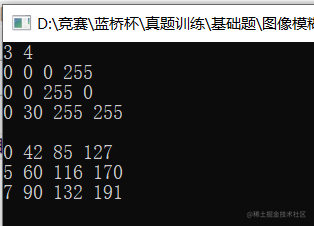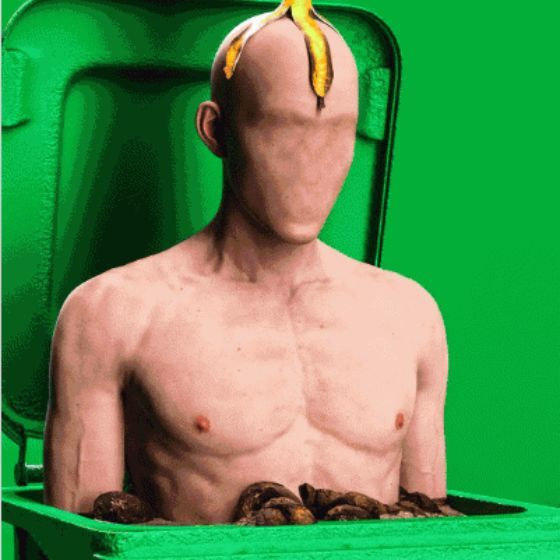# 算法题每日一练 --- 第 4 天：图像模糊问题

• 2022 年 7 月 21 日
• 本文字数：1009 字

阅读完需：约 3 分钟## 二、题目要求

### 考察

1.数组，搜索2.建议用时10~20min

## 三、问题分析

定义一个8*2的数组，int c={0,1,0,-1,-1,0,1,0,1,1,-1,1,-1,-1,1,-1};作为延伸的方向。在for循环中 x=i+c[k]，y=j+c[k]，判断上下左右和其它总共8个方向坐标值。对于x，y的范围x>=1&&x<=n&&y>=1&&y<=m;

## 四、编码实现

#include<iostream>using namespace std;int main(){  int a,b;//定义两个二维数组   int c={0,1,0,-1,-1,0,1,0,1,1,-1,1,-1,-1,1,-1};//方向数组   int n,m,i,j,k;  cin>>n>>m;//输入数据   for(i=1;i<=n;i++)  {    for(j=1;j<=m;j++)    {      cin>>a[i][j];//输入     }  }  int ans,sum=0;//定义，周围的像素和和个数   for(i=1;i<=n;i++)  {    for(j=1;j<=m;j++)    {      sum=a[i][j];//像素值初始化       ans=1;//像素个数初始化       for(k=0;k<8;k++)      {        int x=i+c[k];//x坐标         int y=j+c[k];//y坐标         if(x>=1&&x<=n&&y>=1&&y<=m)//判断坐标是否在范围         {          ans++;//像素个数++           sum+=a[x][y];//像素值++         }      }      b[i][j]=sum/ans;//更新变量值     }  }  for(i=1;i<=n;i++)  {    for(j=1;j<=m;j++)    {      cout<<b[i][j]<<" ";//输出数组     }cout<<"\n";  }  return 0;}

## 五、输出结果## 评论